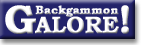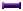TheoryForum Archive : Theory

 Drop rate on initial doubles

 From: Gary Wong Address: gary@cs.arizona.edu Date: 29 July 1998 Subject: Re: Cube theory question Forum: rec.games.backgammon Google: wtiukgqzdc.fsf@brigantine.CS.Arizona.EDU

```Phill Skelton writes:
> Does anyone have any idea roughly what fraction of doubles should be
> taken or dropped? I know this is a pretty vague question, so I'll
> try to explain what I mean.

Actually I think it's a nice and precise question -- especially compared
to a few of the others that have been asked here recently :-)

(WARNING: Boring mathematical content included.  Persons of a
boredom-sensitive or non-mathematically inclined nature should
pain persists.)

> Given that large swings in equity can occur in a few rolls, it is
> (as we all know) possible to go from not being good enough to double,
> through to a double/drop position in just one roll per player. In
> what fraction of games does this occur? I am asking because I have
> been analysing my results recently,and I am looking for evidence that
> I am on average doubling too early (more doubles taken by my opponents,
> less dropped) or too late (more doubles dropped) on average. Do any
> of our resident experts have any feeling for what these numbers ought
> to be?

I'm more of a resident than an expert, but I'm going to inflict my
earlier, in trying to present a reasonable model of the distribution
of points won per game.  In the end I decided it was too hard to
reason about exactly, gave up, and was going to assume an empirically
derived take/drop ratio and see whether that assumption led to a
reasonable agreement with observations of points won in real games.

I don't have a complete answer, but here's some thoughts that might
situations (they're `easy' to decide anyway, simply by enumerating all
36 or 1296 dice rolls over the next exchange).  Ignore gammons, to
keep things simple.  Assume both players are `perfect'.  Assume you're
making an initial double, not a redouble (you're more reluctant to
double if you already own the cube, so P would be higher in that case).

The question we now ask is: Assume that it is correct to double this
turn.  What is the probability P that it is also correct to drop?

Since you have the opportunity to double, we know you didn't double
last turn.  And since we're also assuming perfect play, we know it
would have been _incorrect_ to double then.  Since we're not considering
gammons, the only reason it wouldn't have been correct to double
was that your position was not good enough.  Therefore, the position
last turn was no double/take, and this turn is double/(we don't know).

To be a take last turn, we know your equity E then must have been less
than your opponent's drop point D.  In practice D will vary slightly
from roll to roll (since it depends on the recube vig, which in turn
depends on the cube efficiency, which varies...) but let's ignore that
for now.

To be a double this turn, we know your cubeful equity after doubling
immediately must be higher that your cubeful equity from holding the
cube for one more turn and then deciding.  This is loosely equivalent
to claiming that the cost of doubling now (and then rolling junk and
not losing your market after all) is less than the cost of _not_
doubling (and then rolling well and losing your market).  Calculating
these costs is a complicated question in itself, but let's go with
the conventional rule-of-thumb for now and assume (given volatility V)
you double if E + V > D, and your opponent drops if E > D.  The question
we previously asked (given that it wasn't a double last turn, and it
is a double this turn, what is the probability it is now a drop?)
becomes: given E + V <= D, and E' + V' > D, what is the probability
that E' > D?  (E and V are last turn's equity and volatility; E' and
V' are this turn's).  E and E' are obviously related: E' = E + delta,
where delta is assumed to be normally distributed with mean 0 and
standard deviation V.  There are too many inequalities in there for
me, so let's simplify things by making yet another assumption.  Assume
that last roll, it was _almost_ a double (E + V = D).  It's obviously
easier to lose your market from that position than from being nowhere
near a double, so any conclusion we make will be an upper bound on P.
From the definition of E', P( E' > D ) is P( E + delta > D ), and since
we assume E + V = D, that's the same as P( delta > V ).  That's just
the plain old normal distribution P( z > 1 ), which is about 0.16.
However, we haven't yet taken into account that we also assumed that
E' + V' > D.  Since E + V = D, for E' + V' to exceed D then either
E' must be greater than E; V' must be greater than V; or both.  Measuring
the change in volatility gets pretty messy, so let's just assume that
E' must exceed E.  Under a normal distribution this will happen exactly
half the time, so although the probability of losing your market is
only 0.16, the probability of losing your market _given that your position
improved_ is twice that, or 0.32.  Remember the assumption we made in
the middle of this paragraph will tend to overestimate P, so we
conclude that the probability that any particular double should be
dropped is no more than 32%.  The other assumption we made was to
ignore the change in volatility -- unfortunately there's little you
anyway!  We measure the equity, and call the variance in equity the
volatility.  What do we call the variance in volatility?)  It depends
on the position -- for instance, in prime-vs-prime games the
volatility is very high, and it is often correct to lose your market
without doubling (ie. P will be higher).  In long races, the
volatility is very low, and with correct play you should get the
opportunity to make an efficient double (ie. P will be lower).  The
overall P depends on the relative probability of these situations
occurring.  Our conclusion must be that the _average_ P is under 32%,
but P will vary from position to position.

Well, that's quite a long-winded non-answer :-)  But I hope the
reasoning behind it helps in any case.  I feel kind of cruel making
you read all that without giving you some sort of independent
verification that I'm vaguely on the right track, so here goes: a few
years ago, Stig Eide posted an article with statistics from 1000 games
of Jellyfish playing itself.  Among the results was that 35% of games
resulted in a single point win -- assuming the only way you can win a
single point is for your opponent to drop an initial double, that
would indicate that 35% of doubles ought to be dropped.  This is a
little higher than our theoretical P of 32%, so it looks as if
some of our assumptions led us to underestimate P slightly, or
Jellyfish drops slightly more doubles than it should, or it waits
slightly too long before doubling, or that sometimes it is correct to
win a single point without ever turning the cube, or the difference
can be attributed to sampling error in the experiment.

(NB: Boring mathematical content ends.)

Whether you believe the wobbly acalephoid computerised fish or me, it
looks as if P ~= 1/3 (2 takes to every drop) is in the right ballpark.

Cheers,
Gary.
--
Gary Wong, Department of Computer Science, University of Arizona
gary@cs.arizona.edu     http://www.cs.arizona.edu/~gary/
```

### Theory

Derivation of drop points  (Michael J. Zehr, Apr 1998)
Double/take/drop rates  (Gary Wong, June 1999)
Drop rate on initial doubles  (Gary Wong, July 1998)
Error rate--Why count forced moves?  (Ian Shaw+, Apr 2009)
Error rates--Repeated ND errors  (Joe Russell+, July 2009)
Inconsistencies in how EMG equity is calculated  (Jeremy Bagai, Nov 2007)Janowski's formulas  (Joern Thyssen+, Aug 2000)
Janowski's formulas  (Stig Eide, Sept 1999)
Jump Model for money game cube decisions  (Mark Higgins+, Mar 2012)
Number of distinct positions  (Walter Trice, June 1997)
Number of no-contact positions  (Darse Billings+, Mar 2004)
Optimal strategy?  (Gary Wong, July 1998)
Proof that backgammon terminates  (Robert Koca+, May 1994)Solvability of backgammon  (Gary Wong, June 1998)
Undefined equity  (Paul Tanenbaum+, Aug 1997)Under-doubling dice  (Bill Taylor, Dec 1997)Variance reduction  (Oliver Riordan, July 2003)From GammOnLineLong messageRecommended readingRecent addition

 Book Suggestions Books Cheating Chouettes Computer Dice Cube Handling Cube Handling in Races Equipment Etiquette Extreme Gammon Fun and frustration GNU Backgammon History Jellyfish Learning Luck versus Skill Magazines & E-zines Match Archives Match Equities Match Play Match Play at 2-away/2-away Miscellaneous Opening Rolls Pip Counting Play Sites Probability and Statistics Programming Propositions Puzzles Ratings Rollouts Rules Rulings Snowie Software Source Code Strategy--Backgames Strategy--Bearing Off Strategy--Checker play Terminology Theory Tournaments Uncategorized Variations